# Chessboards, Implicit Expansion, REPELEM, and Unicode Chess Queens

A few weeks ago, I wrote about a solver algorithm for the Eight Queens Problem. The post included diagrams like this one.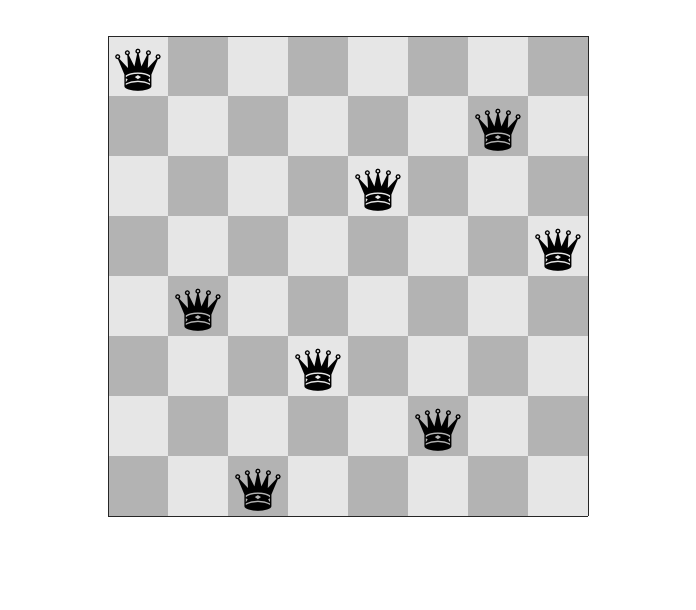Today I want to show you how I made that diagram in MATLAB. First, let's talk about the board. Back in 2011, I wrote about a variety of ways to make a checkerboard (or chessboard) pattern. In that post, I played games with repmat, powers of -1, floor, and round. It got a little crazy.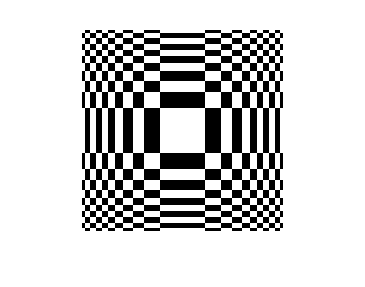I'm still fond of using integer powers of -1, like this.

$(-1)^{(n_1 + n_2)}$

In 2011, I used ndgrid to make a matrix of integer powers, $n_1 + n_2$.

[n1,n2] = ndgrid(0:4);
(-1).^(n1 + n2)

ans =

1    -1     1    -1     1
-1     1    -1     1    -1
1    -1     1    -1     1
-1     1    -1     1    -1
1    -1     1    -1     1



Since the introduction of implicit expansion in R2016b, though, I no longer need to use ndgrid to explicitly form the matrix of powers.

n = 0:4;
(-1).^(n + n')

ans =

1    -1     1    -1     1
-1     1    -1     1    -1
1    -1     1    -1     1
-1     1    -1     1    -1
1    -1     1    -1     1



In my eight queens code, here's how I got the shades of gray for a chessboard. (There are lots of ways to do this.)

N = 8;

dark_square_color = .7;
light_square_color = .9;

color_range = light_square_color - dark_square_color;
f = (-1).^((1:N)' + (1:N));
f = (f + 1) * color_range / 2;
f = f + dark_square_color;
f(1:4,1:4)

ans =

0.9000    0.7000    0.9000    0.7000
0.7000    0.9000    0.7000    0.9000
0.9000    0.7000    0.9000    0.7000
0.7000    0.9000    0.7000    0.9000



Next, I needed to replicate each element of f to make an image with larger squares. Do you know how to replicate elements of a matrix? Some experienced MATLAB users would do it using the kron function. Now, however, you can just use the new repelem function. The reference page tells you when this function was introduced.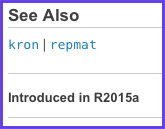I'm going to make my squares 60 pixels wide, and then I'll turn it into a truecolor image so that the pixel colors are independent of the figure's colormap.

pixel_size = 60;
f = repelem(f,pixel_size,pixel_size);
f = repmat(f,1,1,3);
h = imshow(f,'InitialMagnification',100);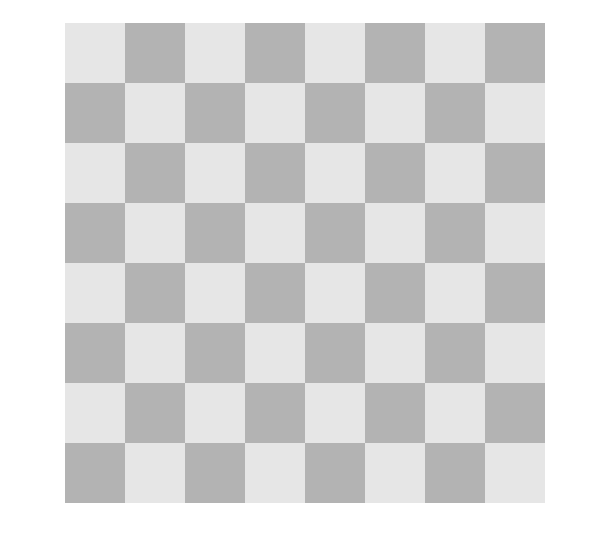Now I want to show you a coordinate system trick. If you turn on the axes display, you can see the image pixel coordinates. (In Image Processing Toolbox terminology, these are called intrinsic coordinates.)

axis on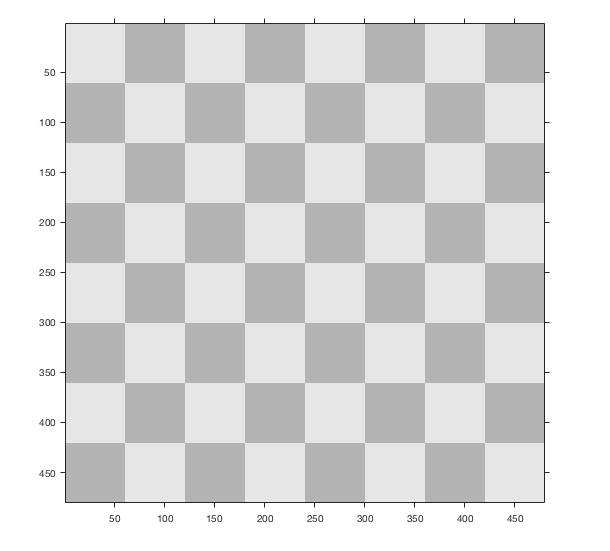When I get to the part about displaying the queens, however, I would like to be able to place them based on the coordinates of each chessboard square, independent of the number of pixels per square. I can do that by manipulating the XData and YData properties of the image. These properties assign spatial coordinates to the left/right and top/bottom edges of the image.

h.XData = [0.5 N+0.5];
h.YData = [0.5 N+0.5];
axis([0.5 N+0.5 0.5 N+0.5])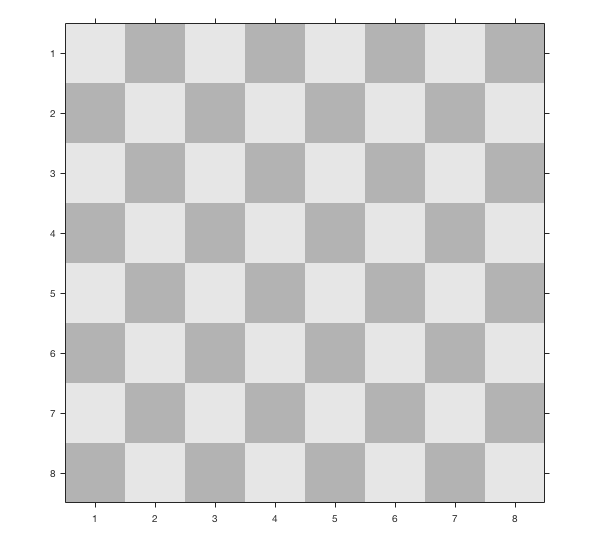Now let's put some queens on the board. This turns out to be pretty easy because you can just do it with a text object. Since R2014b, you've been able to draw text in MATLAB graphics using Unicode characters. And the Unicode character set includes chess symbols! The black queen symbol is at code point 9819 (decimal).

queen = char(9819)

queen =

'♛'



Let's put a queen on the square in the second row, third column. (That's $x=3$, $y=2$.)

hq = text(3,2,queen);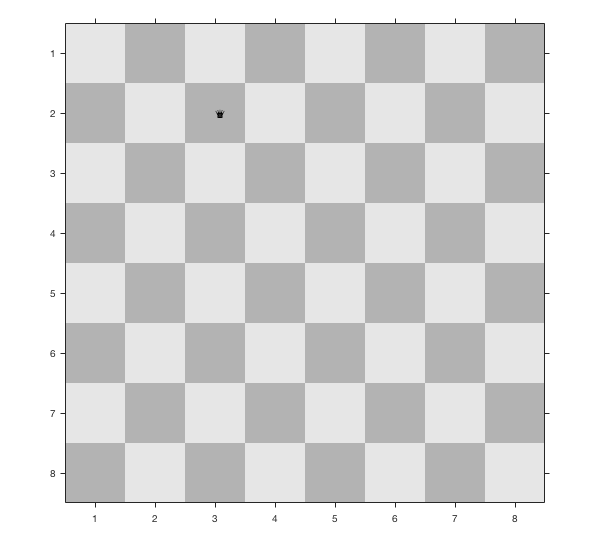Oops, our queen is pretty small, and she also appears to be off-center. Let's fix that.

hq.FontSize = 40;
hq.HorizontalAlignment = 'center';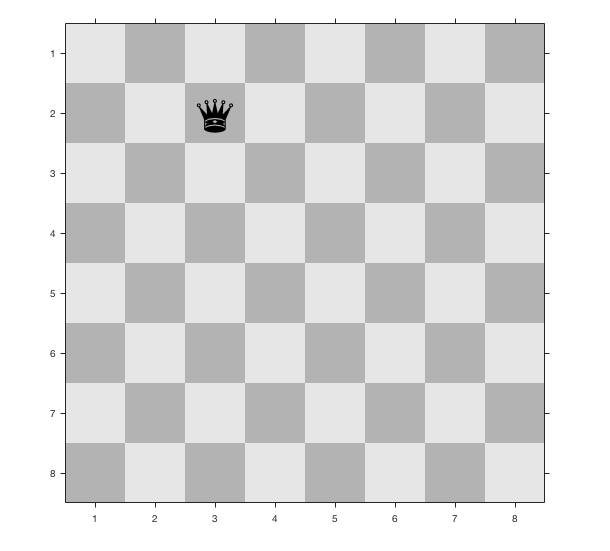That's better.

I have one more little coding trick to show you. When I wrote my eight queens animation code, I wanted to have an easy to show and remove a queen at any square on the board. So, I made an matrix of text objects, one at each square. Then I could just index into the matrix of text objects and turn the Visible property on and off. Here's how. (First, let me delete the text object I just made.)

delete(hq)
for r = 1:N
for c = 1:N
queens(r,c) = text(c,r,char(9819),...
'HorizontalAlignment','center',...
'Visible','off',...
'FontSize',40);
end
end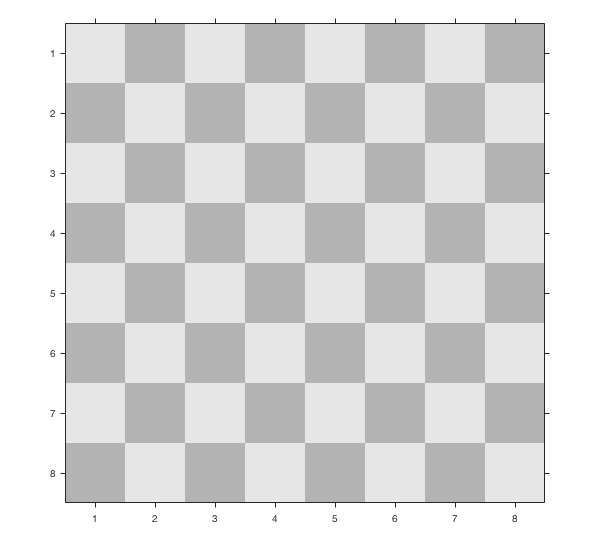OK, let's turn on the queen displays on a couple of squares.

queens(5,3).Visible = 'on';
queens(2,4).Visible = 'on';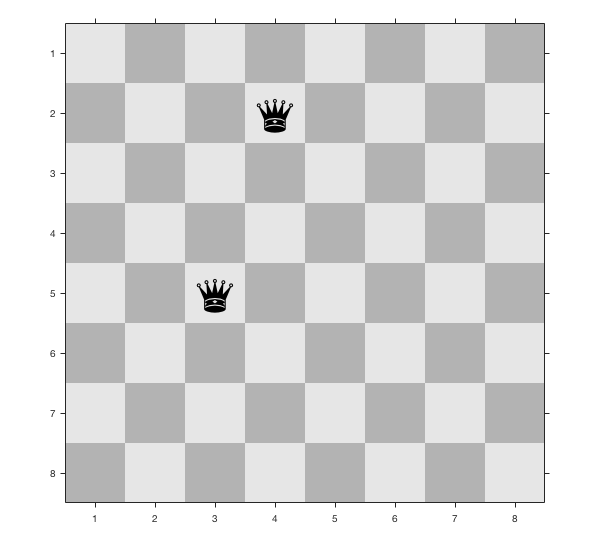For a final bit of fun, the function set lets you modify properties of a whole array of graphics objects at once.

Turn them all on!

set(queens,'Visible','on')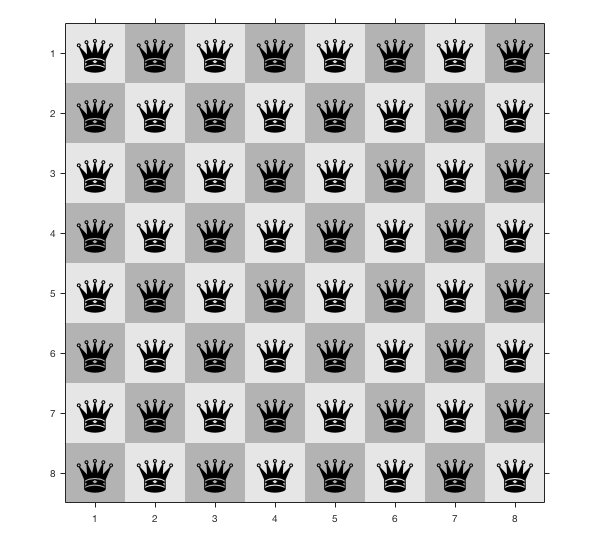(The board above, by the way, is NOT a solution to the Eight Queens Problem. In case you were wondering.)

Published with MATLAB® R2017a

|

### 댓글

댓글을 남기려면 링크 를 클릭하여 MathWorks 계정에 로그인하거나 계정을 새로 만드십시오.